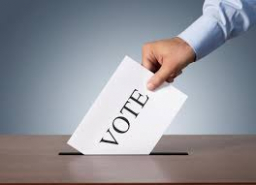# Yarhands

Yarhands statistical consult conducted statistical analysis on the election results from 1992 to 2012 to assess the chances of the NPP winning the election in Ghana's Volta region. The research reveals that 15% of inhabitants in the Volta region favor the NPP. A random sample of 200 inhabitants was selected from the Volta region, and each person was asked which party they would vote for in the 2016 general election. What is the probability that:

I) exactly two persons will vote for NPP?
II) at least three people will vote for NPP.
III) fewer than two persons will vote for NPP?

a =  0
b =  1
c =  0

### Step-by-step explanation:Did you find an error or inaccuracy? Feel free to write us. Thank you!

Tips for related online calculators
Looking for a statistical calculator?
Our percentage calculator will help you quickly calculate various typical tasks with percentages.
Would you like to compute the count of combinations?